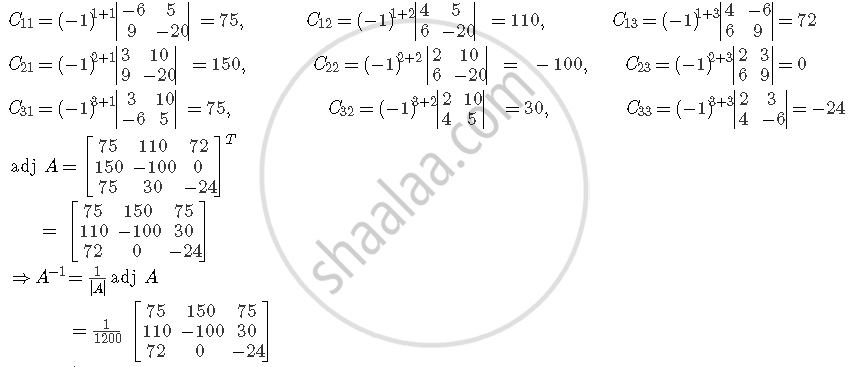Share

# If a = Matrix ((2,3,10),(4,-6,5),(6,9,-20)), Find A Power (-1). Using Apower(-1) Solve the System of Equation 2byx + 3byy +10/Z = 2;4by - 6byy + 5byz = 5; 6byx + 9byy - 20byz = -4 - CBSE (Commerce) Class 12 - Mathematics

#### Question

if A =  ((2,3,10),(4,-6,5),(6,9,-20)), Find A^(-1). Using A^(-1) Solve the system of equation 2/x + 3/y +10/z = 2; 4/x - 6/y + 5/z = 5; 6/x + 9/y - 20/z = -4

#### Solution

Let 1/x be a, 1/y be b and 1/x be c

Here

A = [(2,3,10),(4,-6,5),(6,9,-20)] and B = [(2),(5),(-4)]

|A| = [(2,3,10),(4,-6,5),(6,9,-20)]

= 2(120 - 45) -3 (-80-30) +10 (36 + 36)

= 150 + 330 + 720

= 1200

Let C_(ij) be the cofactors of the elements a_(ij) in A[a_(ij)] ThenX = A^(-1)B

=>[(a),(b),(c)] = 1/1200 [(75,150,75),(110,-100,30),(72,0,-24)][(2),(5),(-4)]

=> [(a),(b),(c)] = 1/1200 [(150+750-300),(220-500-120),(144+0+96)]

=> [(a),(b),(c)] = 1/1200 [(600),(-400),(240)]

=> [(a),(b),(c)] = [(1/2),(1/(-3)), (1/5)]

=> x = 1/a = 2, y = 1/b = -3, " and " z = 1/c = 5

∴ x = 2, y = 3 and z = 5

Is there an error in this question or solution?

#### Video TutorialsVIEW ALL 

Solution If a = Matrix ((2,3,10),(4,-6,5),(6,9,-20)), Find A Power (-1). Using Apower(-1) Solve the System of Equation 2byx + 3byy +10/Z = 2;4by - 6byy + 5byz = 5; 6byx + 9byy - 20byz = -4 Concept: Minors and Co-factors.
S